### Coordinate Geometry - Solutions

CBSE Class 9 Mathematics

NCERT Solutions
CHAPTER 3
Coordinate Geometry
(Ex. 3.1)

1. How will you describe the position of a table lamp on your study table to another person?

Ans. Let us consider the given below figure of a study stable, on which a study lamp is placed.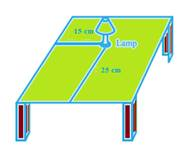Let us consider the lamp on the table as a point and the table as a plane. From the figure, we can conclude that the table is rectangular in shape, when observed from the top. The table has a short edge and a long edge.

Let us measure the distance of the lamp from the shorter edge and the longer edge. Let us assume that the distance of the lamp from the shorter edge is 15 cm and from the longer edge, its 25 cm.

Therefore, we can conclude that the position of the lamp on the table can be described in two ways depending on the order of the axes as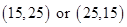.

2. (Street Plan): A city has two main roads which cross each other at the centre of the city. These two roads are along the North-South direction and East-West direction.

All the other streets of the city run parallel to these roads and are 200 m apart. There are 5 streets in each direction. Using 1cm = 200 m, draw a model of the city on your notebook. Represent the roads/streets by single lines.

There are many cross- streets in your model. A particular cross-street is made by two streets, one running in the North - South direction and another in the East – West direction. Each cross street is referred to in the following manner: If the 2nd street running in the North - South direction and 5th in the East - West direction meet at some crossing, then we will call this cross-street (2, 5). Using this convention, find:

(i) how many cross - streets can be referred to as (4, 3).
(ii) how many cross - streets can be referred to as (3, 4).

Ans. We need to draw two perpendicular lines as the two main roads of the city that cross each other at the center and let us mark it as N-S and E-W.
Let us take the scale as 1 cm = 200m.
We need to draw five streets that are parallel to both the main roads, to get the given below figure.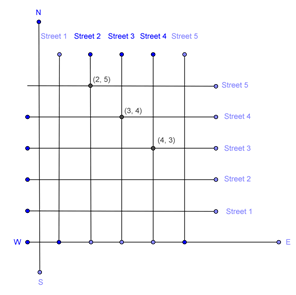(i) From the figure, we can conclude that only one point have the coordinates as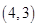.
Therefore, we can conclude that only one cross - street can be referred to as (4, 3).

(ii) From the figure, we can conclude that only one point have the coordinates as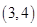.
Therefore, we can conclude that only one cross - street can be referred to as (3, 4).